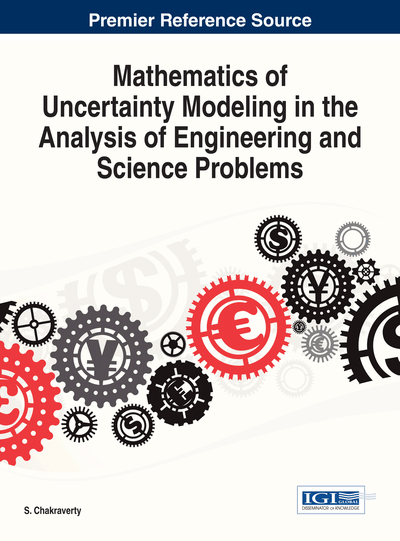# Construction of Normal Fuzzy Numbers using the Mathematics of Partial Presence

Hemanta K. Baruah (Gauhati University, India)
DOI: 10.4018/978-1-4666-4991-0.ch006

## Abstract

Every normal law of fuzziness can be expressed in terms of two laws of randomness defined in the measure theoretic sense. Indeed, two probability measures are necessary and sufficient to define a normal law of fuzziness. Hence, the measure theoretic matters with reference to fuzziness have to be studied accordingly. In this chapter, we are going to discuss how to construct normal fuzzy numbers using this concept which is based on our mathematics of partial presence. Three case studies have been presented with reference to expressing stock prices in terms of fuzzy numbers. We have shown how exactly a normal fuzzy number appears.
Chapter Preview
Top

## 1. Introduction

The introduction of the theory of fuzzy sets was a paradigm changing event in the history of mathematics. After it was applied in certain fields, it never had to look back. A lot of software based on fuzziness has meanwhile been made available, and the application oriented people have been applying such software in all sorts of fields.

In most of the applications, whenever one finds that a situation is fuzzy, one uses the triangular fuzzy number. The triangular fuzzy number is the simplest of all fuzzy numbers, and it is easy to deal with computationally. Other types of fuzzy numbers have also been prescribed. However, the mathematical reason why a particular type of fuzzy number should be used in any situation, and why other types of fuzzy numbers are unsuitable for that situation, has not yet been mathematically explained. In this chapter, we are going to put forward a discussion on how to construct normal fuzzy numbers using our mathematics of partial presence. From our case studies, it became evident that the triangular fuzzy number appears to be a very natural choice to represent fuzziness.

It was observed that not all uncertainties can be explained using the theory of probability, and that there are situations which can be explained by the mathematics of fuzziness (Zadeh, 1965). Soon thereafter, Zadeh (1968) propounded an idea of establishing a law of probability defined on a space on which a law of fuzziness had already been defined with a view to finding some sort of consistency between the two laws. However, first if we say that the theory of probability is unable to explain a situation, and that the mathematics of fuzziness would be appropriate to deal with it, and then if we proceed to find consistency between the concerned law of fuzziness and a law of probability that has been defined on the same interval, we would anyway be contradicting ourselves. If a situation is fuzzy, it cannot be probabilistic. On the other hand, if a situation is probabilistic, the notion of fuzziness need not enter into that. For this, one has to understand whether the concept of fuzziness or the concept of probability is actually fit to explain the uncertainty involved in a situation, and hence one has to understand whether a probability density function or a fuzzy membership function should come into the picture. How to construct a probability density function is well known. How to construct a membership function was however never given much importance. The researchers just assume a membership function heuristically, and continue to apply fuzziness.

Zadeh (1968) noted that the notions of an event and its probability can be extended in a natural fashion to fuzzy events. Indeed, he has mentioned that a fuzzy variable is associated with a possibility distribution in a similar way in which a random variable is associated with a probability distribution. Further, he hypothesized that for the same element in a given interval, there can be a fuzzy membership value as well as a probability mass (Zadeh, 1978). He however pointed out that his probability-possibility consistency principle is not a precise law, declaring the principle heuristic thereby. This is a very important point to be noted.

As Zadeh himself commented that his principle was not a precise law, other such principles had started to come into the picture. Klir’s (1990) view in this regard was to extract a law of probability by scaling a law of fuzziness defined in a given interval. In other words, even when a variable is fuzzy, and not probabilistic, Klir’s principle was to define a probability law from the fuzzy law concerned. In effect, this was nothing but a process of normalizing the values of membership to get probability mass so that the total probability is equal to 1.

## Complete Chapter List

Search this Book:
Reset